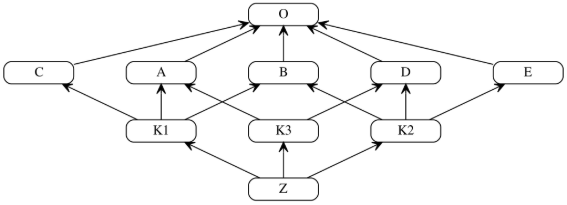# Python 多重继承问题之 MRO 和 C3 算法

0收藏

C3算法实现了三种重要特性：

1. 保持继承拓扑图的一致性。
2. 保证局部优先原则（比如A继承C，C继承B，那么A读取父类方法，应该优先使用C的方法而不是B的方法）。
3. 保证单调性原则（即子类不改变父类的方法搜索顺序）。

C3主要用于在多继承时判断继承调用的路径(来自于哪个类)。在Python2.3之前是基于深度优先算法，为了解决原来基于深度优先搜索算法不满足本地优先级，和单调性以及继承不清晰的问题，从Python2.3起应用了新的C3算法。 在Python官网的The Python 2.3 Method Resolution Order中作者举了例子，说明这一情况。

``````F=type('Food', (), {remember2buy:'spam'})
G=type('GoodFood', (F,E), {})``````

C3算法简介

``class B(A)``

``class B(A1,A2,...,An)``

``mro(B) = [B] + merge(mro(A1), mro(A2),...,mro(An), [A1,A2,...,An])``

merge操作是C3算法的核心，可以递归运算。

``````class A(object):pass
class B(object):pass
class C(object):pass
class E(A,B):pass
class F(B,C):pass
class G(E,F):pass``````

``````mro(E) = [E] + merge(mro(A), mro(B), [A,B])
= [E] + merge([A,O], [B,O], [A,B])``````

``mro(E) = [E,A] + merge([O], [B,O], [B])``

``````mro(E) = [E,A,B] + merge([O], [O])
= [E,A,B,O]``````

``````mro(F) = [F] + merge(mro(B), mro(C), [B,C])
= [F] + merge([B,O], [C,O], [B,C])
= [F,B] + merge([O], [C,O], [C])
= [F,B,C] + merge([O], [O])
= [F,B,C,O]

mro(G) = [G] + merge(mro[E], mro[F], [E,F])
= [G] + merge([E,A,B,O], [F,B,C,O], [E,F])
= [G,E] + merge([A,B,O], [F,B,C,O], [F])
= [G,E,A] + merge([B,O], [F,B,C,O], [F])
= [G,E,A,F] + merge([B,O], [B,C,O])
= [G,E,A,F,B] + merge([O], [C,O])
= [G,E,A,F,B,C] + merge([O], [O])
= [G,E,A,F,B,C,O]``````

Wiki有一个Python版本的C3算法:

``````def c3MRO(cls):
if cls is object:
# 讨论假设顶层基类为object，递归终止
return [object]

# 构造C3-MRO算法的总式，递归开始
mergeList = [c3MRO(baseCls) for baseCls in cls.__bases__]
mergeList.append(list(cls.__bases__))
mro = [cls] + merge(mergeList)
return mro

def merge(inLists):
if not inLists:
# 若合并的内容为空，返回空list
# 配合下文的排除空list操作，递归终止
return []

# 遍历要合并的mro
for mroList in inLists:
### 但按照多继承中地基础规则（一个类只能被继承一次），
for cmpList in inLists[inLists.index(mroList) + 1:]:
break
else:
nextList = []
for mergeItem in inLists:
if mergeItem:
# 排除空list
nextList.append(mergeItem)
# 递归开始
else:
raise TypeError``````

``````class A(object):pass
class B(object):pass
class C(object):pass
class E(A,B):pass
class F(B,C):pass
class G(E,F):pass

print([i.__name__ for i in c3MRO(G)]) ## ['G', 'E', 'A', 'F', 'B', 'C', 'object']``````

``````class Type(type):
def __repr__(cls):
return cls.__name__

A = Type('A', (object,), {})
B = Type('B', (object,), {})
C = Type('C', (object,), {})
D = Type('D', (object,), {})
E = Type('E', (object,), {})
K1 = Type('K1', (A, B, C), {})
K2 = Type('K2', (D, B, E), {})
K3 = Type('K3', (D, A), {})
Z = Type('Z', (K1, K2,K3), {})````````````print(Z.mro()) # [Z, K1, K2, K3, D, A, B, C, E, <class 'object'>]

print([i.__name__ for i in c3MRO(Z)])
# ['Z', 'K1', 'K2', 'K3', 'D', 'A', 'B', 'C', 'E', 'object']``````帖子
视频
声望
粉丝
相关问题
社区精华内容